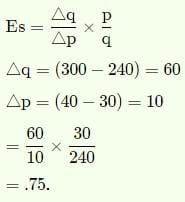# Test: Theory Of Supply

## 30 Questions MCQ Test Crash Course of Micro Economics -Class 12 | Test: Theory Of Supply

Description
Attempt Test: Theory Of Supply | 30 questions in 30 minutes | Mock test for CA Foundation preparation | Free important questions MCQ to study Crash Course of Micro Economics -Class 12 for CA Foundation Exam | Download free PDF with solutions
QUESTION: 1

### If the price of apples rises from Rs. 30 per kg to Rs. 40 per kg and the supply increases from 240 kg to Rs. 300 kg. Elasticity of supply is

Solution:QUESTION: 2

Solution:
QUESTION: 3

### When supply price increase in the short run, the profit of the producer________:

Solution:
QUESTION: 4

The supply of a goods refers to:

Solution:
QUESTION: 5

When change in the quantity supplied is proportionate to the change in the price, the producer is said to have ______:

Solution:
QUESTION: 6

Increase or Decrease in supply means

Solution:
QUESTION: 7

Short run price is also called by the name of ________.

Solution:
QUESTION: 8

Price of a product falls by 10% and its demand rises by 30%. The elasticity of demand is

Solution:

Price of a product falls by 10% and its demand rises by 30%. The elasticity of demand is 3.

QUESTION: 9

Increase or decrease in supply means:

Solution:
QUESTION: 10

When supply is perfectly inelastic, elasticity of supply is equal to:

Solution:
QUESTION: 11

A perfectly inelastic supply curve will be

Solution:

Perfectly Inelastic Supply
In other words, the supply of such a commodity always remains constant no matter what the price is. A perfectly inelastic supply is represented as Es= 0. Further, a perfectly inelastic supply curve is a vertical straight line parallel to the Y-axis.

QUESTION: 12

A change in the supply of a commodity along with same supply curve may occur due to:

Solution:
QUESTION: 13

If a 20% fall in price brings about a 10% fall in quantity supplied, in such a case elasticity of supply will be equal to:

Solution:
QUESTION: 14

Elasticity of supply is defined as responsiveness of quantity supplied of a good to change in _____.

Solution:
QUESTION: 15

If supply curve is Perfectly Inelastic, the supply curve is:

Solution:
QUESTION: 16

If Rs. 20% fall in the price brings about a 10% fall in the quantity supplied, then the elasticity of supply will be equal to:

Solution:
QUESTION: 17

The following are causes of shift in demand EXCEPT the one

Solution:
QUESTION: 18

When Supply Curve shifts to the right there is _____ in Supply.

Solution:
QUESTION: 19

If the supply of a commodity is perfectly elastic, an increase in demand will result in:

Solution:
QUESTION: 20

The price of mangoes increases form Rs. 30 per kilogram to Rs. 40 per kilogram and the supply increases from 240 kilograms the 300 kilograms. What will be the elasticity of supply for mangoes?

Solution:
QUESTION: 21

A horizontal supply curve parallel to the quantity axis implies that the elasticity of supply is

Solution:
QUESTION: 22

If quantity demanded is completely unresponsive to changes in price, demand is:

Solution:

The correct option is D.

Perfectly inelastic demand means that a consumer will buy a good or service regardless of the movement of price. In order for perfectly inelastic demand to exist, it has no close substitutes available.So when a demand for a good is completely unresponsive of the changes in price level this means it is not affected by whatever the price maybe so its perfectly inelastic.

QUESTION: 23

Expansion in supply refers to a situation when the producers are willing to supply a:

Solution:
QUESTION: 24

Increase or Decrease in Supply means:

Solution:

If the supply curve shifts to the right, this is an increase in supply; more is provided for sale at each price. If the supply curve moves inwards, there is a decrease in supply meaning that less will be supplied at each price.

QUESTION: 25

When supply price increase in the short run, the profit of the producer________:

Solution:
QUESTION: 26

When price remains constant and quantity demanded changes, then the elasticity of demand will be:

Solution:
QUESTION: 27

Supply of a commodity is a ________.

Solution:
QUESTION: 28

What is the elasticity of supply, when price changes from Rs. 15 to Rs. 12 and supply change from 6 units to 5 units?

Solution:
QUESTION: 29

At a price of Rs. 25 per kg, the supply of a commodity is 10,000 kg per week. An increase in its price to Rs. 30 per kg, increases the supply of the commodity to 12,000 kg per week. The elasticity of supply will be:-

Solution:
QUESTION: 30

Short run price is also called by the name of ________.

Solution:Use Code STAYHOME200 and get INR 200 additional OFF Use Coupon Code